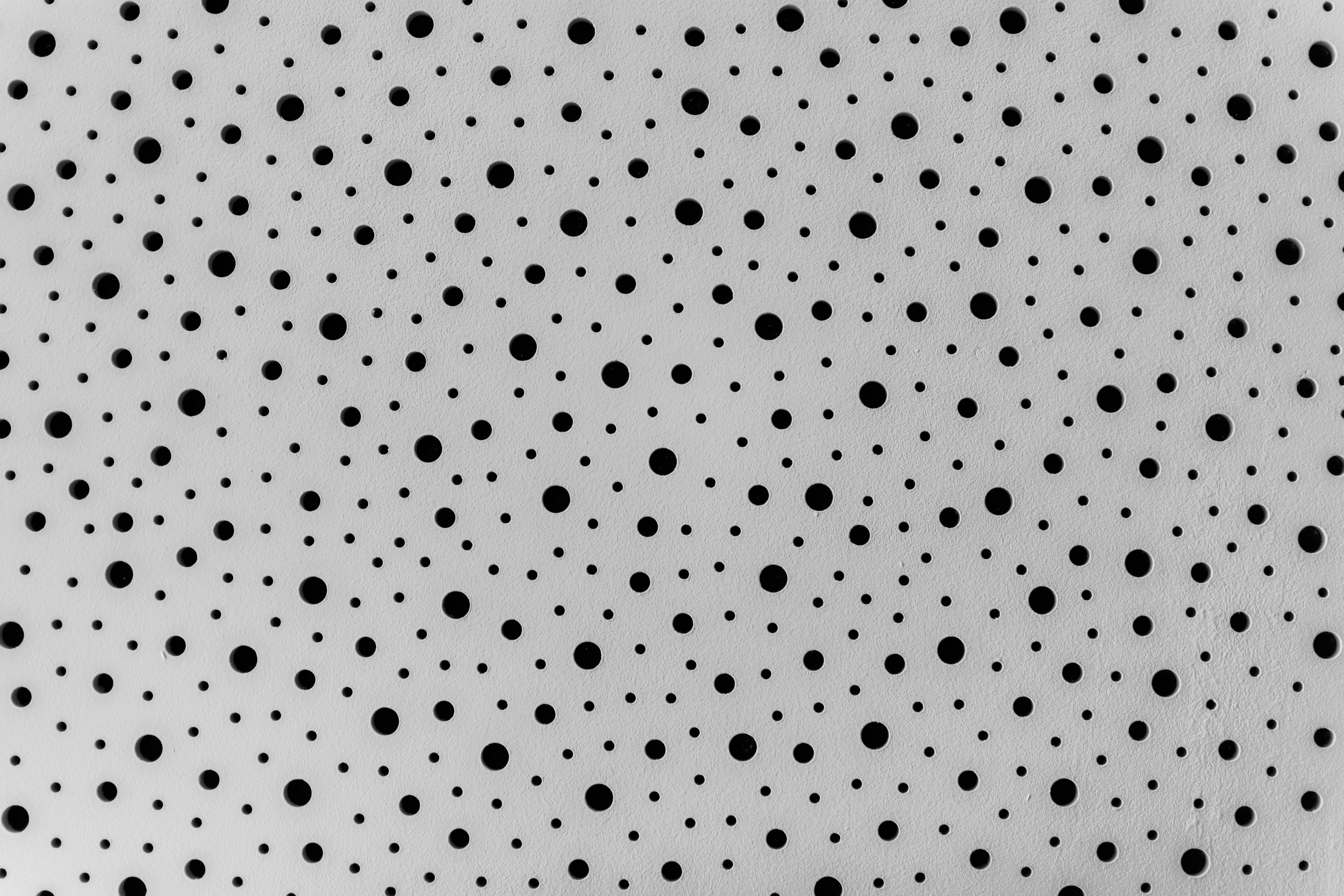# 在 reduce 使用點點點 spread operator 是效能上的 anti-pattern``users.reduce((acc, item) => {  return { ...acc, [item.key]: item.value };}, {});``

``let users = Array.from({ length: 1000 }, (item, i) => ({ key: i, value: i }));console.time();let result = {};users.forEach((acc, item) => {  result[item.key] = item.value;});console.timeEnd();``

``let users = Array.from({ length: 1000 }, (item, i) => ({ key: i, value: i }));console.time();let result = users.reduce((acc, item) => {  return { ...acc, [item.key]: item.value };}, {});console.timeEnd();``

## 替代方案 #

``let users = Array.from({ length: 1000 }, (item, i) => ({ key: i, value: i }));console.time();let result = users.reduce((acc, item) => {  acc[item.key] = item.value;  return acc;}, {});console.timeEnd();``

``let users = Array.from({ length: 1000 }, (item, i) => ({ key: i, value: i }));console.time();let result = Object.fromEntries(users.map((item) => [item.key, item.value]));console.timeEnd();``

## Ref #Jason Chen

Yahoo Taiwan Sr. Frontend Engineer. Write something about web and React.js here.

--